# 15 PARTIAL DERIVATIVES PARTIAL DERIVATIVES 15 3 Partial

• Slides: 96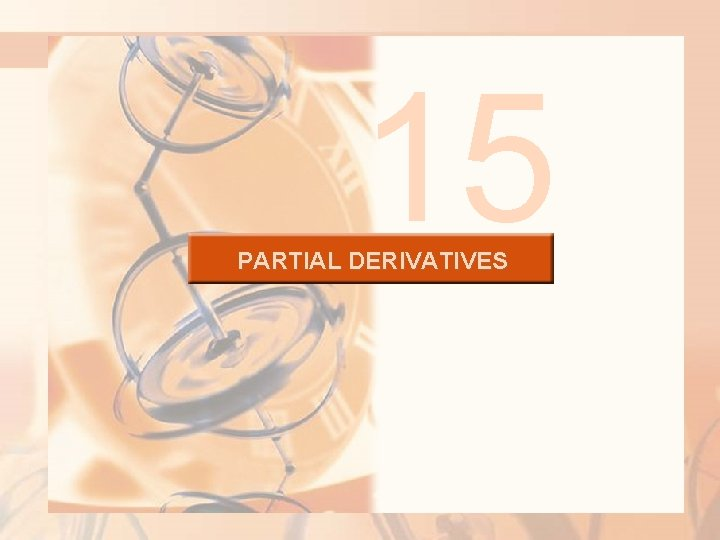15 PARTIAL DERIVATIVES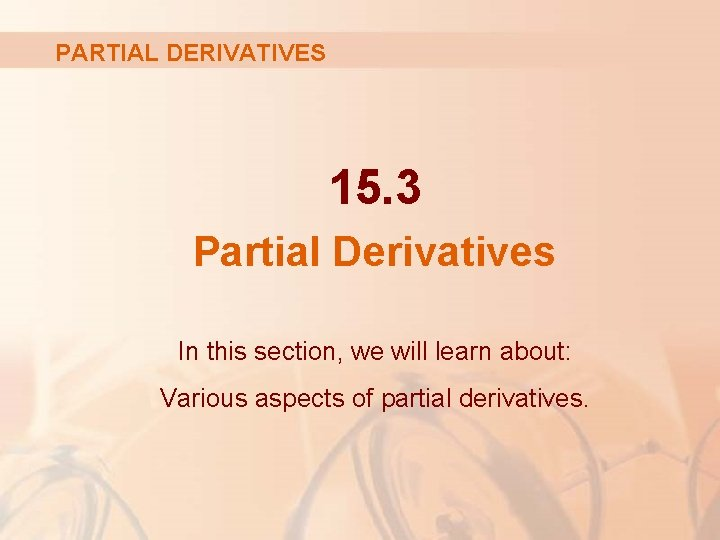PARTIAL DERIVATIVES 15. 3 Partial Derivatives In this section, we will learn about: Various aspects of partial derivatives.INTRODUCTION On a hot day, extreme humidity makes us think the temperature is higher than it really is. In very dry air, though, we perceive the temperature to be lower than thermometer indicates.HEAT INDEX The National Weather Service (NWS) has devised the heat index to describe the combined effects of temperature and humidity. § This is also called the temperature-humidity index, or humidex, in some countries.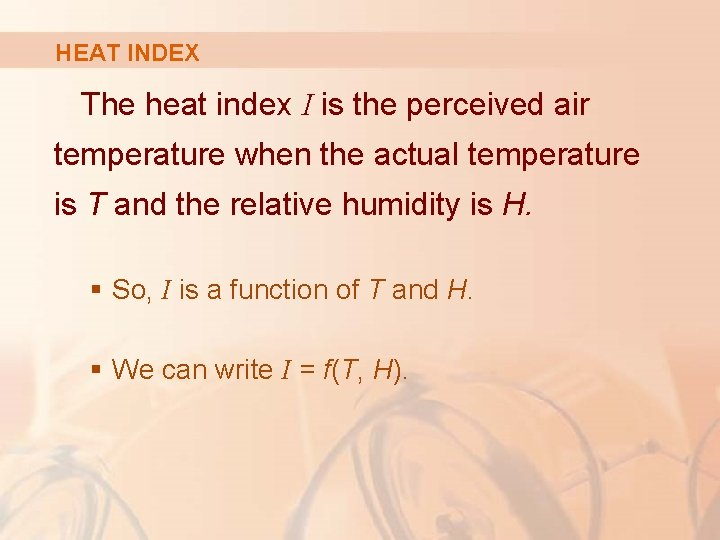HEAT INDEX The heat index I is the perceived air temperature when the actual temperature is T and the relative humidity is H. § So, I is a function of T and H. § We can write I = f(T, H).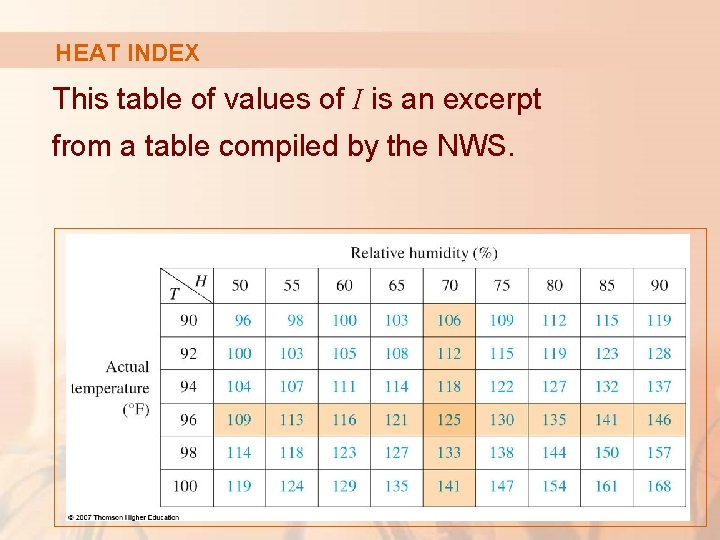HEAT INDEX This table of values of I is an excerpt from a table compiled by the NWS.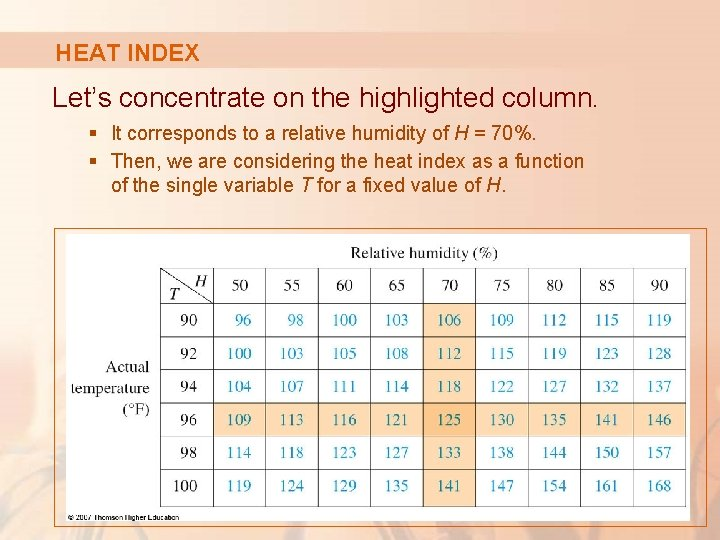HEAT INDEX Let’s concentrate on the highlighted column. § It corresponds to a relative humidity of H = 70%. § Then, we are considering the heat index as a function of the single variable T for a fixed value of H.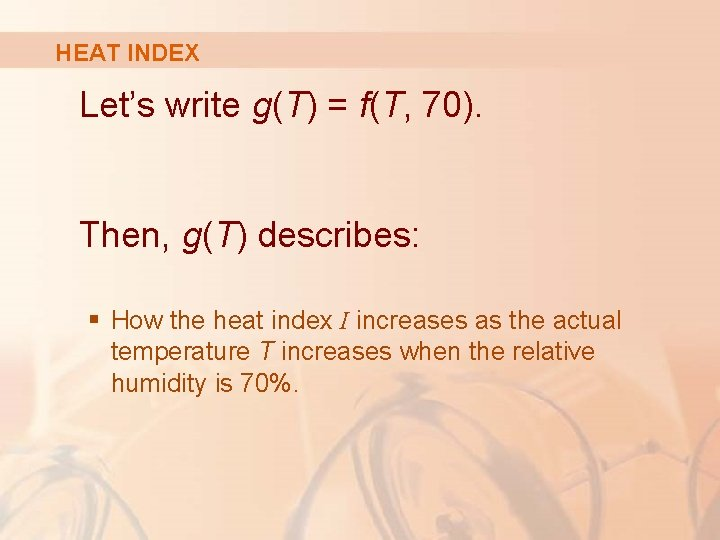HEAT INDEX Let’s write g(T) = f(T, 70). Then, g(T) describes: § How the heat index I increases as the actual temperature T increases when the relative humidity is 70%.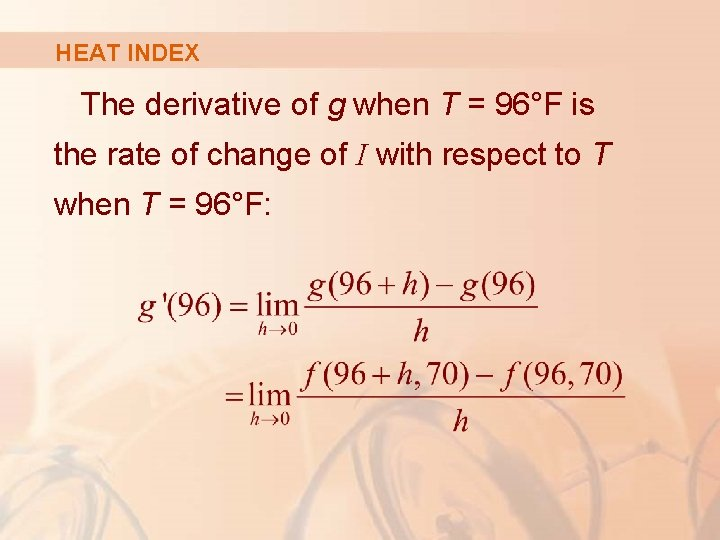HEAT INDEX The derivative of g when T = 96°F is the rate of change of I with respect to T when T = 96°F:HEAT INDEX We can approximate g’(96) using the values in the table by taking h = 2 and – 2.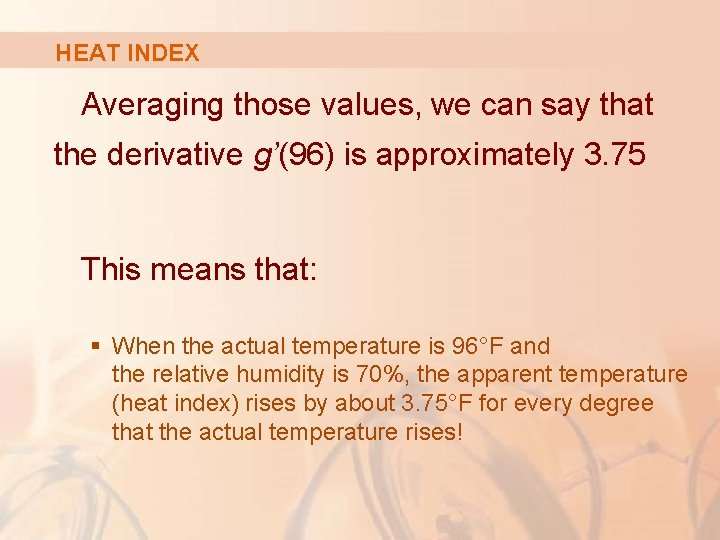HEAT INDEX Averaging those values, we can say that the derivative g’(96) is approximately 3. 75 This means that: § When the actual temperature is 96°F and the relative humidity is 70%, the apparent temperature (heat index) rises by about 3. 75°F for every degree that the actual temperature rises!HEAT INDEX Now, let’s look at the highlighted row. § It corresponds to a fixed temperature of T = 96°F.HEAT INDEX The numbers in the row are values of the function G(H) = f(96, H). § This describes how the heat index increases as the relative humidity H increases when the actual temperature is T = 96°F.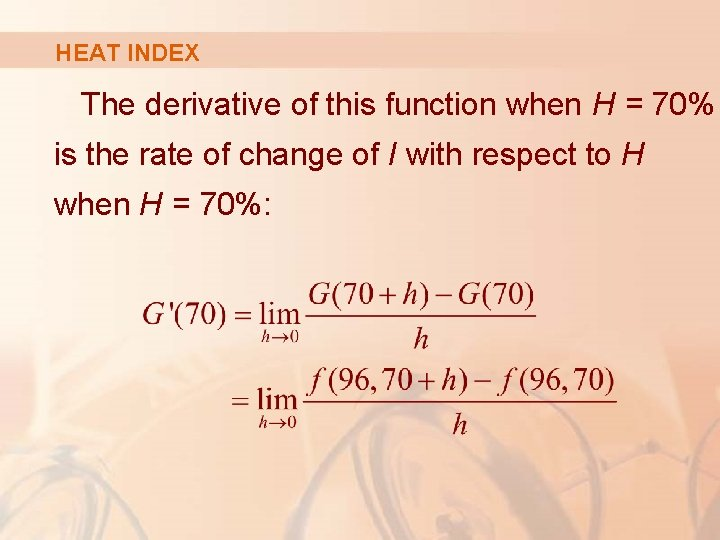HEAT INDEX The derivative of this function when H = 70% is the rate of change of I with respect to H when H = 70%: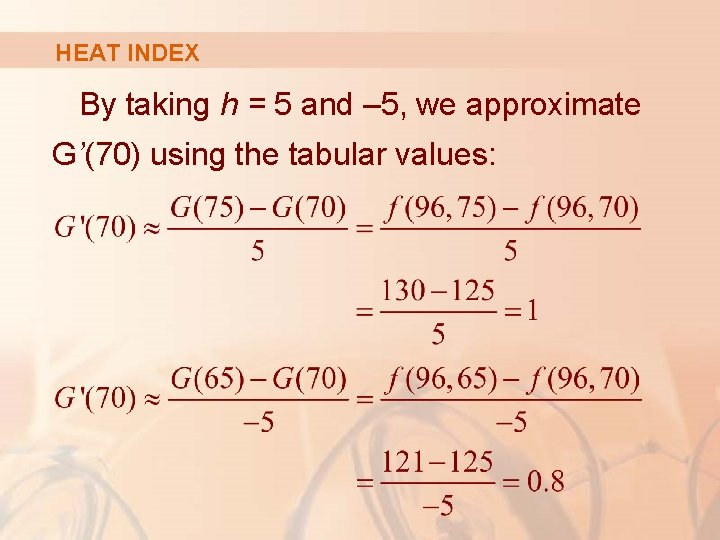HEAT INDEX By taking h = 5 and – 5, we approximate G’(70) using the tabular values:HEAT INDEX By averaging those values, we get the estimate G’(70) ≈ 0. 9 This says that: § When the temperature is 96°F and the relative humidity is 70%, the heat index rises about 0. 9°F for every percent that the relative humidity rises.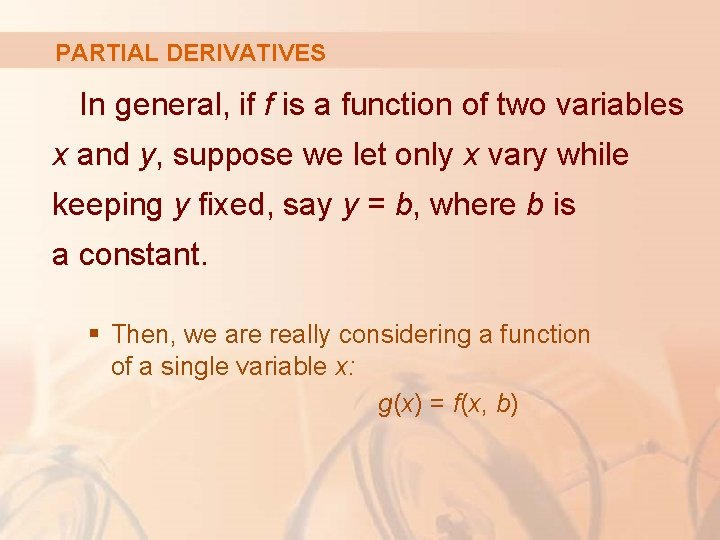PARTIAL DERIVATIVES In general, if f is a function of two variables x and y, suppose we let only x vary while keeping y fixed, say y = b, where b is a constant. § Then, we are really considering a function of a single variable x: g(x) = f(x, b)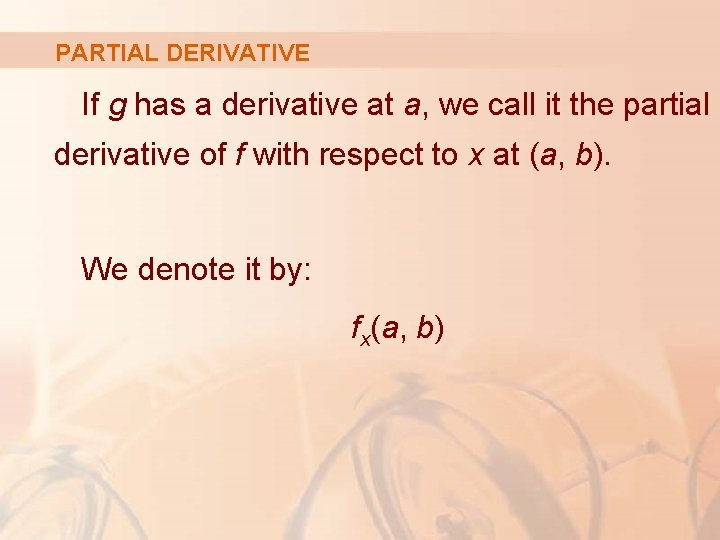PARTIAL DERIVATIVE If g has a derivative at a, we call it the partial derivative of f with respect to x at (a, b). We denote it by: fx(a, b)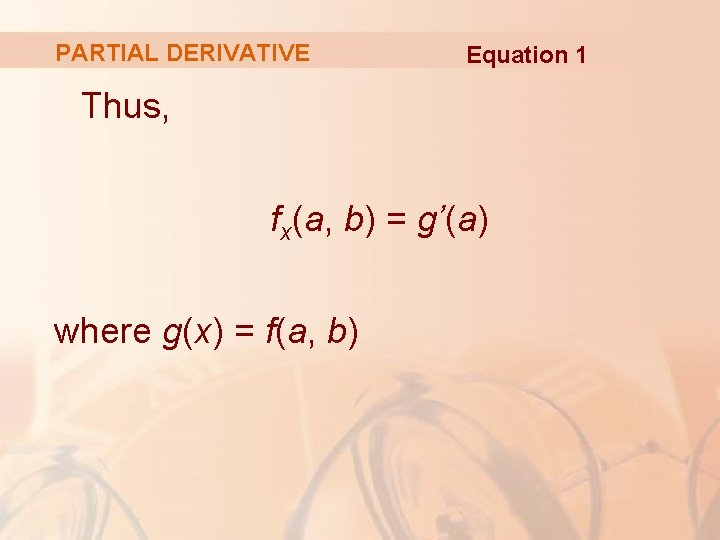PARTIAL DERIVATIVE Equation 1 Thus, fx(a, b) = g’(a) where g(x) = f(a, b)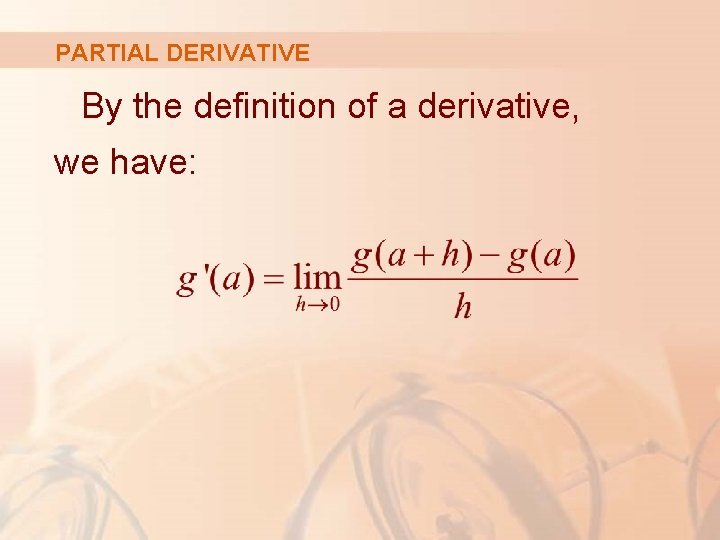PARTIAL DERIVATIVE By the definition of a derivative, we have:PARTIAL DERIVATIVE Equation 2 So, Equation 1 becomes: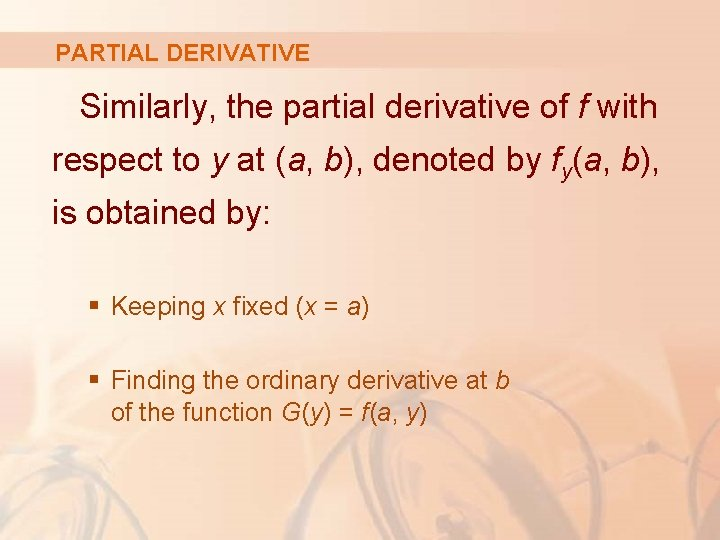PARTIAL DERIVATIVE Similarly, the partial derivative of f with respect to y at (a, b), denoted by fy(a, b), is obtained by: § Keeping x fixed (x = a) § Finding the ordinary derivative at b of the function G(y) = f(a, y)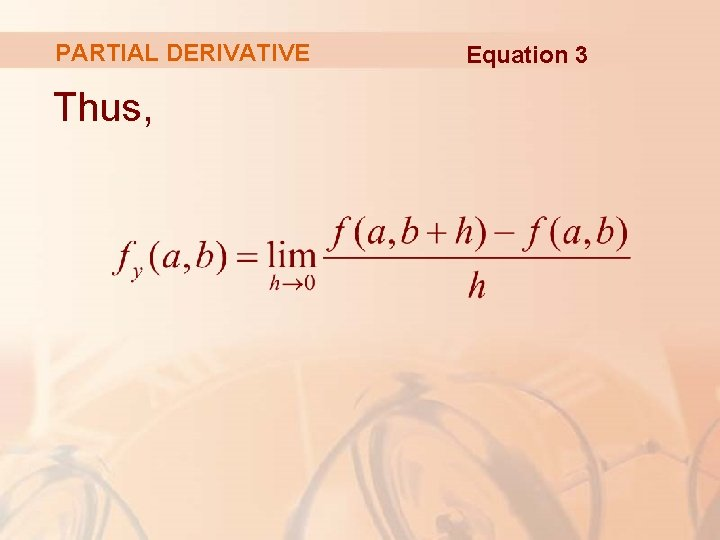PARTIAL DERIVATIVE Thus, Equation 3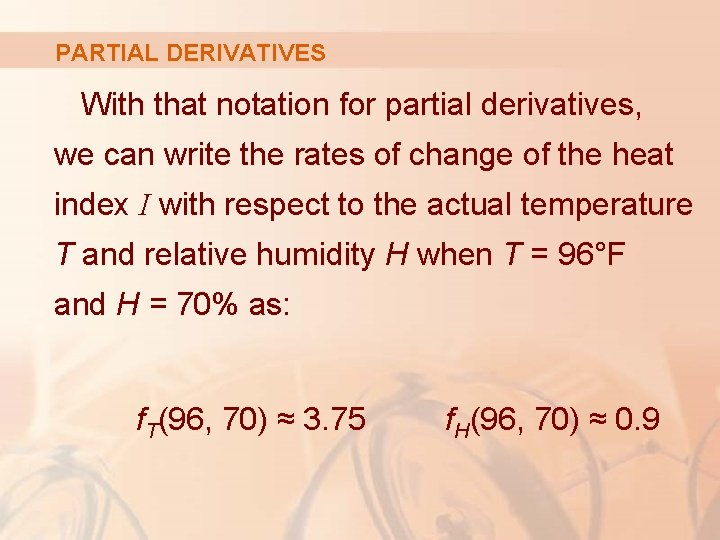PARTIAL DERIVATIVES With that notation for partial derivatives, we can write the rates of change of the heat index I with respect to the actual temperature T and relative humidity H when T = 96°F and H = 70% as: f. T(96, 70) ≈ 3. 75 f. H(96, 70) ≈ 0. 9PARTIAL DERIVATIVES If we now let the point (a, b) vary in Equations 2 and 3, fx and fy become functions of two variables.PARTIAL DERIVATIVES Equations 4 If f is a function of two variables, its partial derivatives are the functions fx and fy defined by:NOTATIONS There are many alternative notations for partial derivatives. § For instance, instead of fx, we can write f 1 or D 1 f (to indicate differentiation with respect to the first variable) or ∂f/∂x. § However, here, ∂f/∂x can’t be interpreted as a ratio of differentials.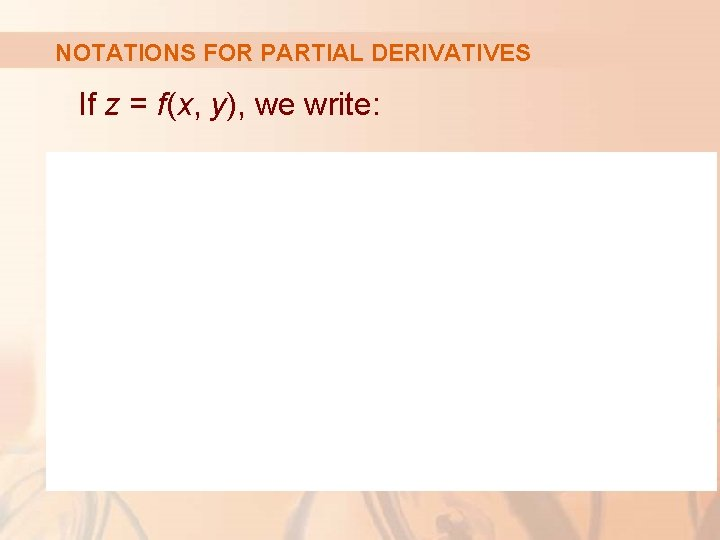NOTATIONS FOR PARTIAL DERIVATIVES If z = f(x, y), we write:PARTIAL DERIVATIVES To compute partial derivatives, all we have to do is: § Remember from Equation 1 that the partial derivative with respect to x is just the ordinary derivative of the function g of a single variable that we get by keeping y fixed.RULE TO FIND PARTIAL DERIVATIVES OF z = f(x, y) Thus, we have this rule. 1. To find fx, regard y as a constant and differentiate f(x, y) with respect to x. 2. To find fy, regard x as a constant and differentiate f(x, y) with respect to y.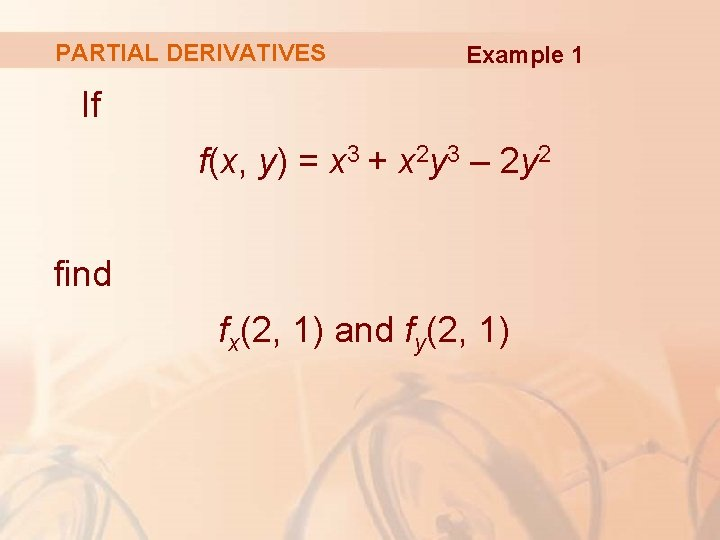PARTIAL DERIVATIVES Example 1 If f(x, y) = x 3 + x 2 y 3 – 2 y 2 find fx(2, 1) and fy(2, 1)PARTIAL DERIVATIVES Example 1 Holding y constant and differentiating with respect to x, we get: fx(x, y) = 3 x 2 + 2 xy 3 § Thus, fx(2, 1) = 3. 22 + 2. 2. 13 = 16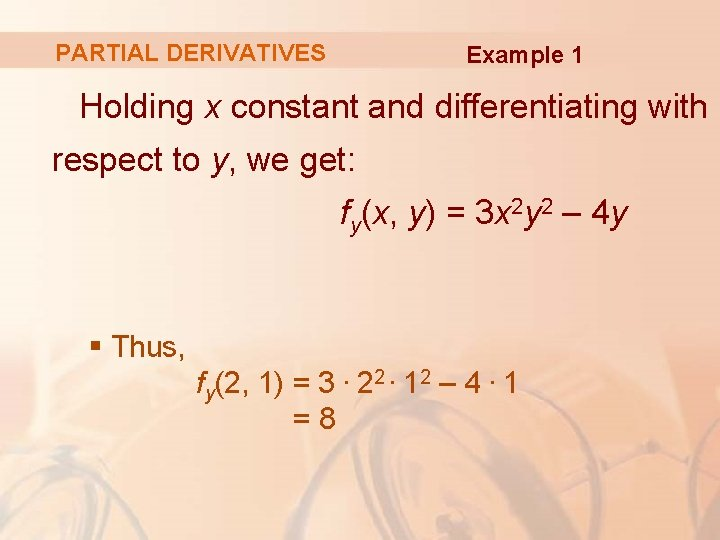PARTIAL DERIVATIVES Example 1 Holding x constant and differentiating with respect to y, we get: fy(x, y) = 3 x 2 y 2 – 4 y § Thus, fy(2, 1) = 3. 22. 12 – 4. 1 =8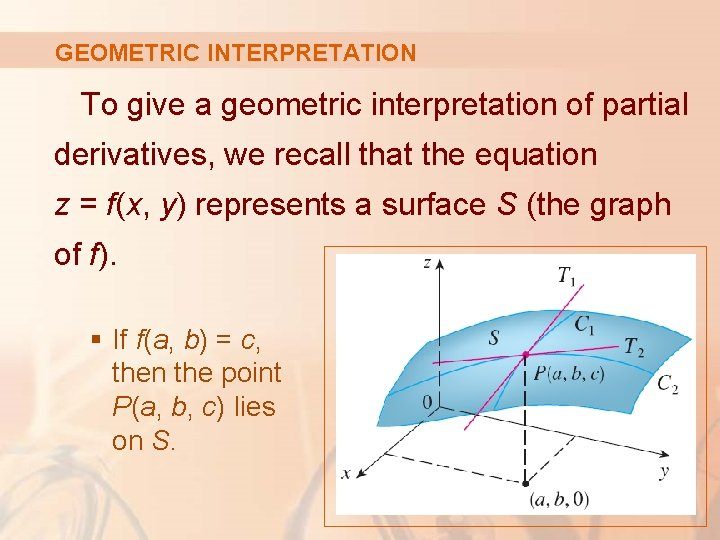GEOMETRIC INTERPRETATION To give a geometric interpretation of partial derivatives, we recall that the equation z = f(x, y) represents a surface S (the graph of f). § If f(a, b) = c, then the point P(a, b, c) lies on S.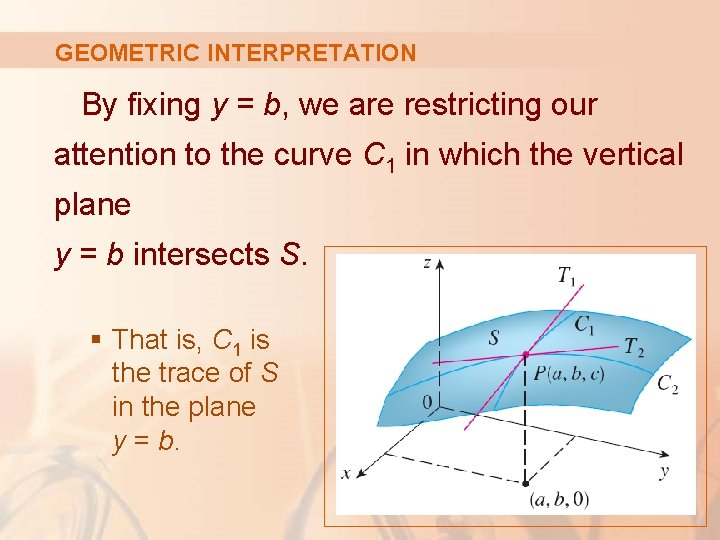GEOMETRIC INTERPRETATION By fixing y = b, we are restricting our attention to the curve C 1 in which the vertical plane y = b intersects S. § That is, C 1 is the trace of S in the plane y = b.GEOMETRIC INTERPRETATION Likewise, the vertical plane x = a intersects S in a curve C 2. Both the curves C 1 and C 2 pass through P.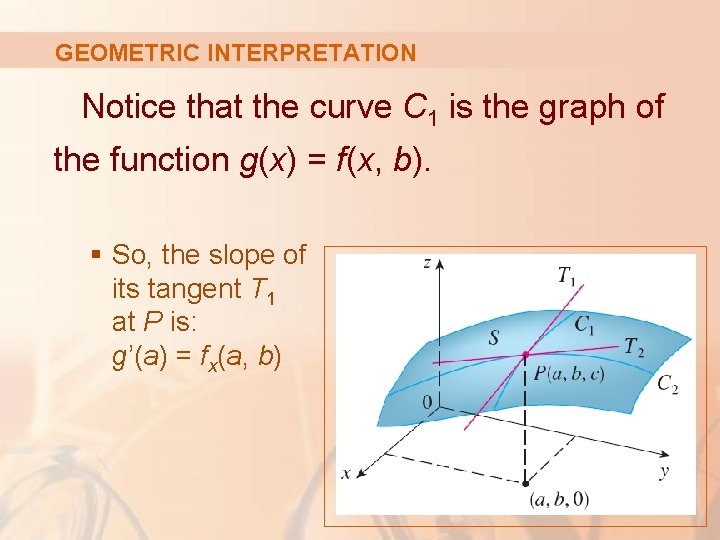GEOMETRIC INTERPRETATION Notice that the curve C 1 is the graph of the function g(x) = f(x, b). § So, the slope of its tangent T 1 at P is: g’(a) = fx(a, b)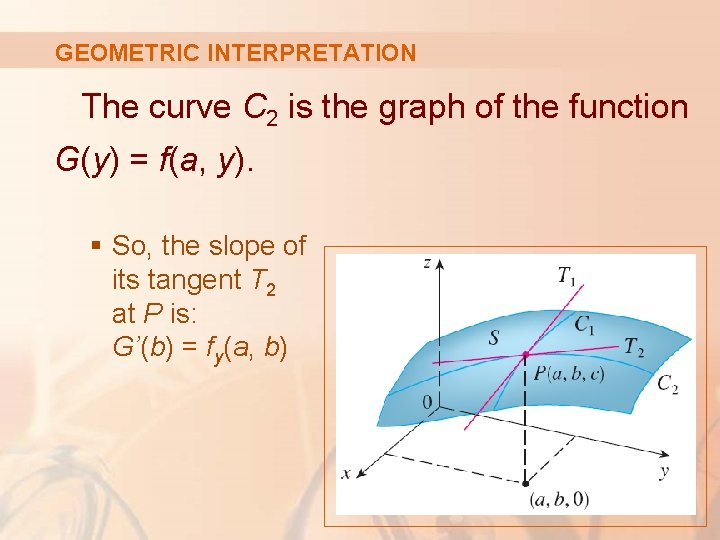GEOMETRIC INTERPRETATION The curve C 2 is the graph of the function G(y) = f(a, y). § So, the slope of its tangent T 2 at P is: G’(b) = fy(a, b)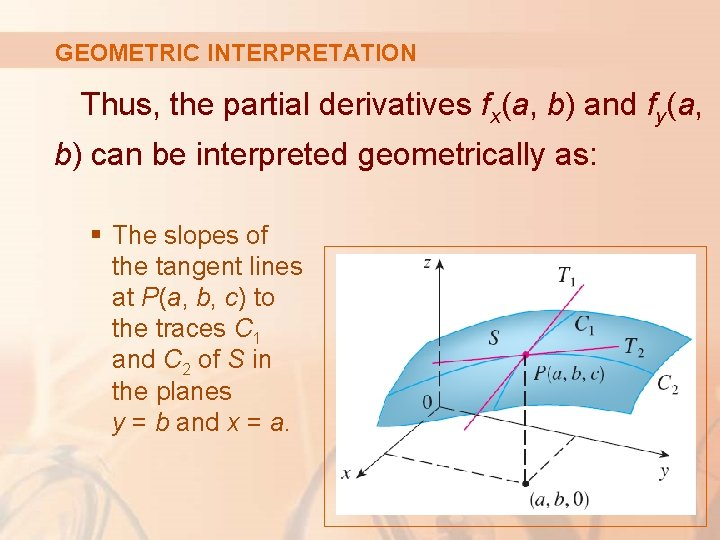GEOMETRIC INTERPRETATION Thus, the partial derivatives fx(a, b) and fy(a, b) can be interpreted geometrically as: § The slopes of the tangent lines at P(a, b, c) to the traces C 1 and C 2 of S in the planes y = b and x = a.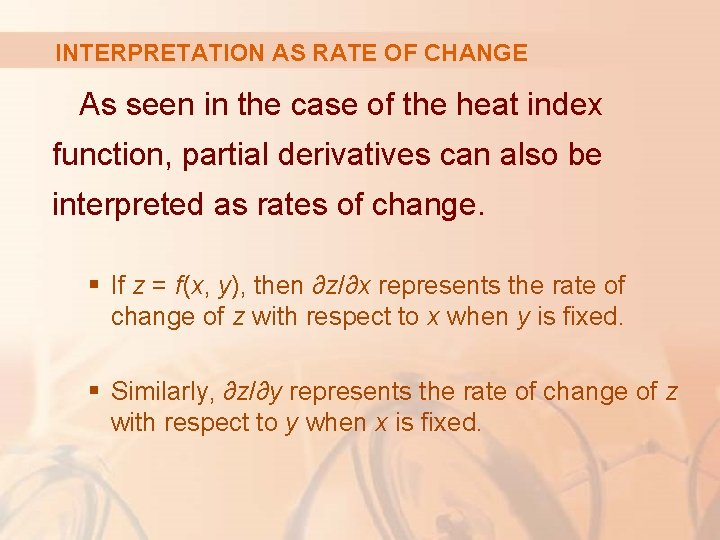INTERPRETATION AS RATE OF CHANGE As seen in the case of the heat index function, partial derivatives can also be interpreted as rates of change. § If z = f(x, y), then ∂z/∂x represents the rate of change of z with respect to x when y is fixed. § Similarly, ∂z/∂y represents the rate of change of z with respect to y when x is fixed.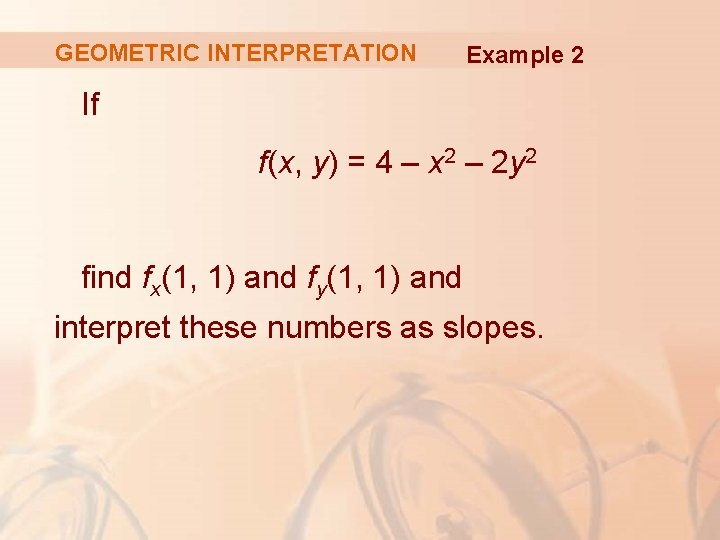GEOMETRIC INTERPRETATION Example 2 If f(x, y) = 4 – x 2 – 2 y 2 find fx(1, 1) and fy(1, 1) and interpret these numbers as slopes.GEOMETRIC INTERPRETATION Example 2 We have: fx(x, y) = -2 x fy(x, y) = -4 y fx(1, 1) = -2 fy(1, 1) = -4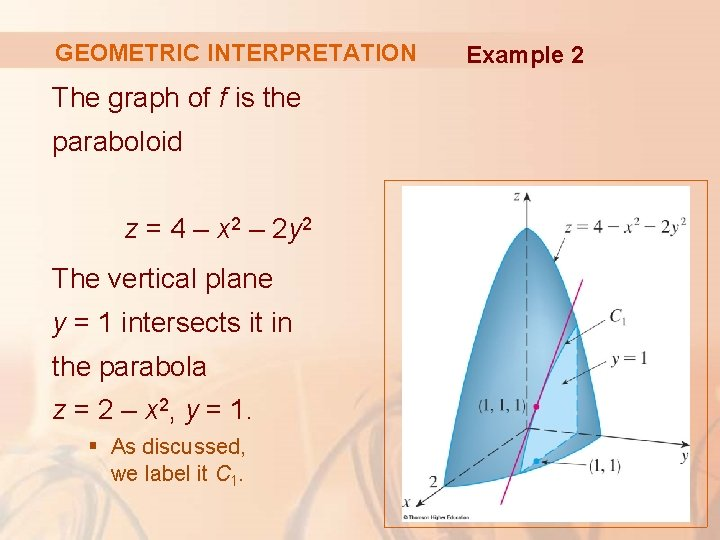GEOMETRIC INTERPRETATION The graph of f is the paraboloid z = 4 – x 2 – 2 y 2 The vertical plane y = 1 intersects it in the parabola z = 2 – x 2, y = 1. § As discussed, we label it C 1. Example 2GEOMETRIC INTERPRETATION The slope of the tangent line to this parabola at the point (1, 1, 1) is: fx(1, 1) = -2 Example 2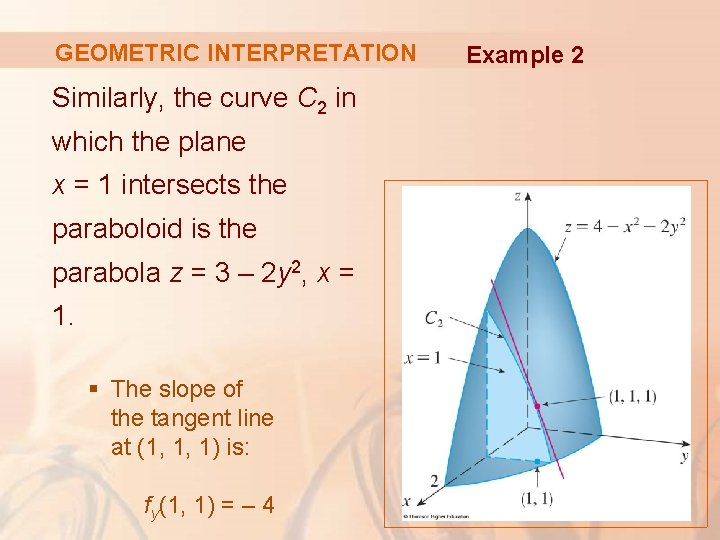GEOMETRIC INTERPRETATION Similarly, the curve C 2 in which the plane x = 1 intersects the paraboloid is the parabola z = 3 – 2 y 2, x = 1. § The slope of the tangent line at (1, 1, 1) is: fy(1, 1) = – 4 Example 2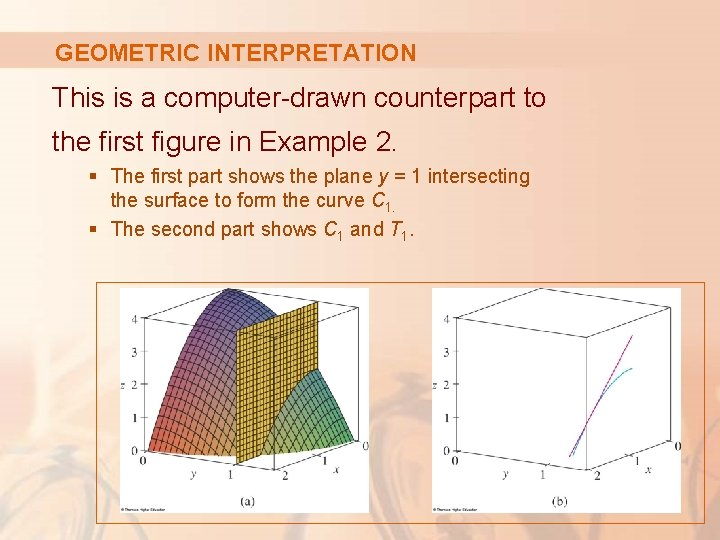GEOMETRIC INTERPRETATION This is a computer-drawn counterpart to the first figure in Example 2. § The first part shows the plane y = 1 intersecting the surface to form the curve C 1. § The second part shows C 1 and T 1.GEOMETRIC INTERPRETATION We have used the vector equations: § r(t) = <t, 1, 2 – t 2> for C 1 § r(t) = <1 + t, 1, 1 – 2 t> for T 1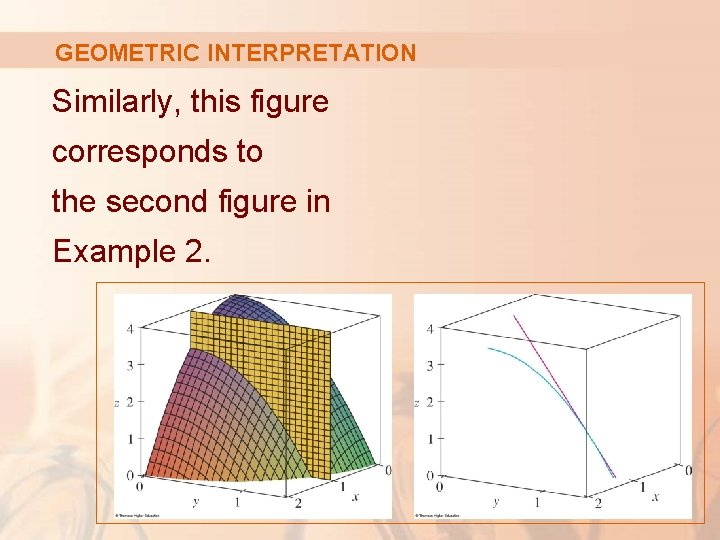GEOMETRIC INTERPRETATION Similarly, this figure corresponds to the second figure in Example 2.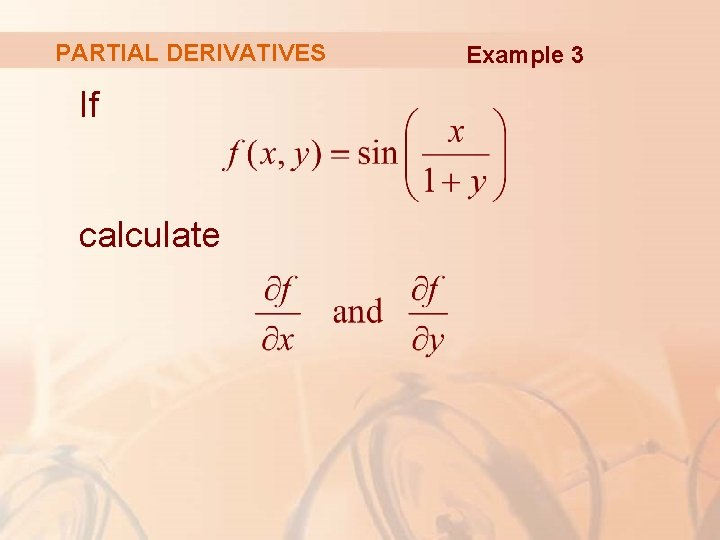PARTIAL DERIVATIVES If calculate Example 3PARTIAL DERIVATIVES Example 3 Using the Chain Rule for functions of one variable, we have:PARTIAL DERIVATIVES Example 4 Find ∂z/∂x and ∂z/∂y if z is defined implicitly as a function of x and y by the equation x 3 + y 3 + z 3 + 6 xyz = 1PARTIAL DERIVATIVES Example 4 To find ∂z/∂x, we differentiate implicitly with respect to x, being careful to treat y as a constant: § Solving for ∂z/∂x, we obtain: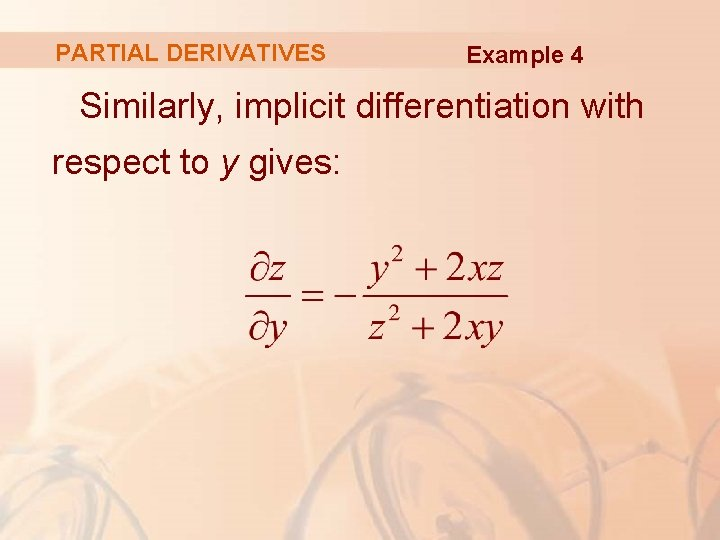PARTIAL DERIVATIVES Example 4 Similarly, implicit differentiation with respect to y gives:PARTIAL DERIVATIVES Some computer algebra systems can plot surfaces defined by implicit equations in three variables. § The figure shows such a plot of the surface defined by the equation in Example 4.FUNCTIONS OF MORE THAN TWO VARIABLES Partial derivatives can also be defined for functions of three or more variables. § For example, if f is a function of three variables x, y, and z, then its partial derivative with respect to x is defined as:FUNCTIONS OF MORE THAN TWO VARIABLES It is found by: § Regarding y and z as constants. § Differentiating f(x, y, z) with respect to x.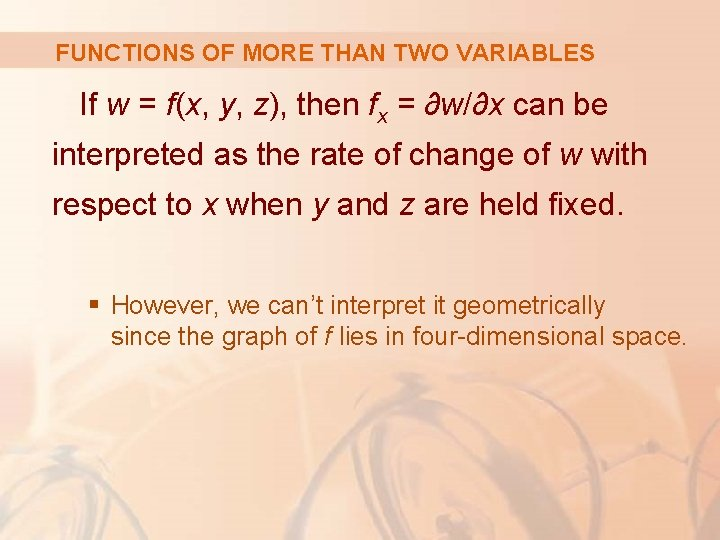FUNCTIONS OF MORE THAN TWO VARIABLES If w = f(x, y, z), then fx = ∂w/∂x can be interpreted as the rate of change of w with respect to x when y and z are held fixed. § However, we can’t interpret it geometrically since the graph of f lies in four-dimensional space.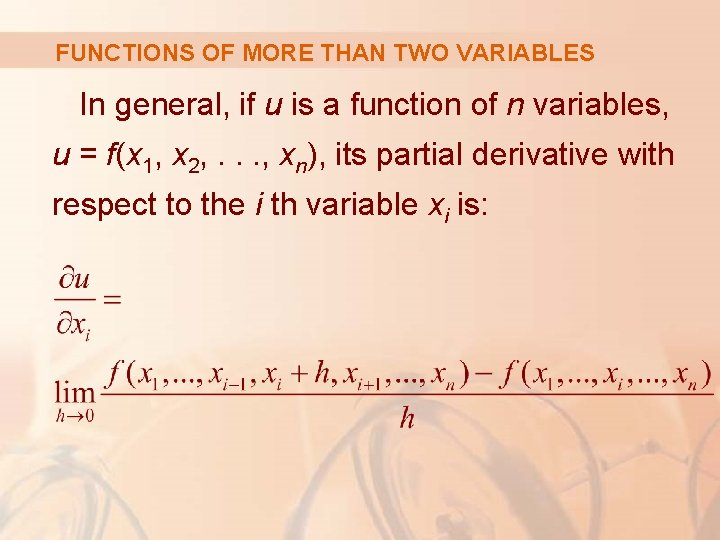FUNCTIONS OF MORE THAN TWO VARIABLES In general, if u is a function of n variables, u = f(x 1, x 2, . . . , xn), its partial derivative with respect to the i th variable xi is: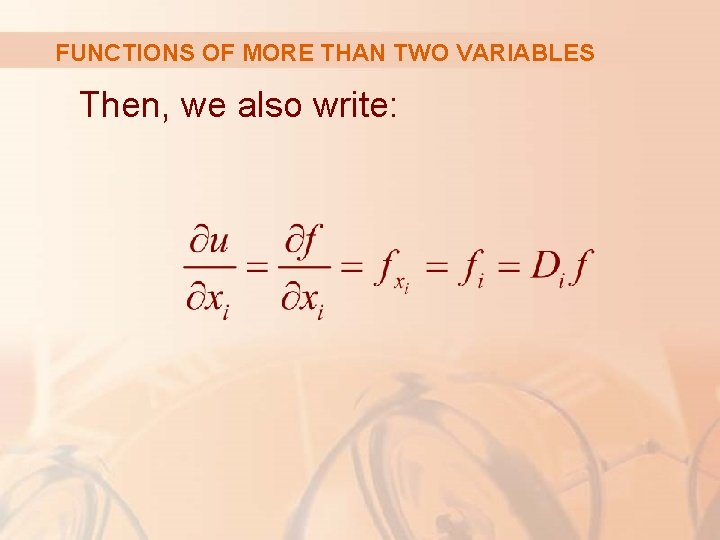FUNCTIONS OF MORE THAN TWO VARIABLES Then, we also write: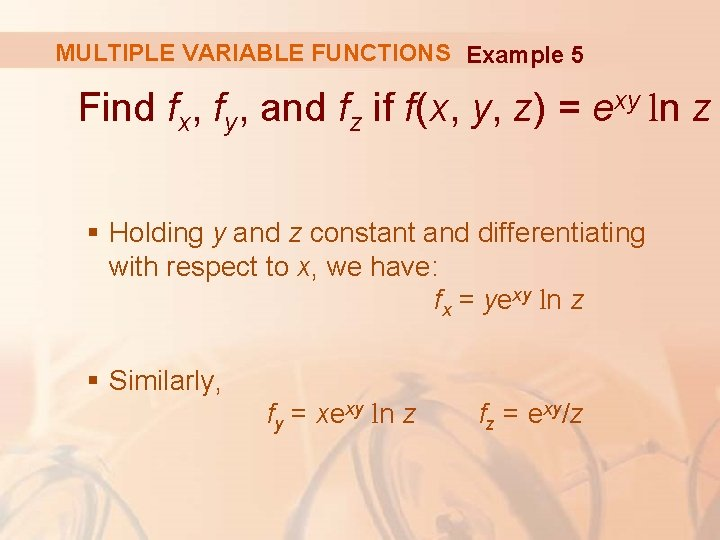MULTIPLE VARIABLE FUNCTIONS Example 5 Find fx, fy, and fz if f(x, y, z) = exy ln z § Holding y and z constant and differentiating with respect to x, we have: fx = yexy ln z § Similarly, fy = xexy ln z fz = exy/z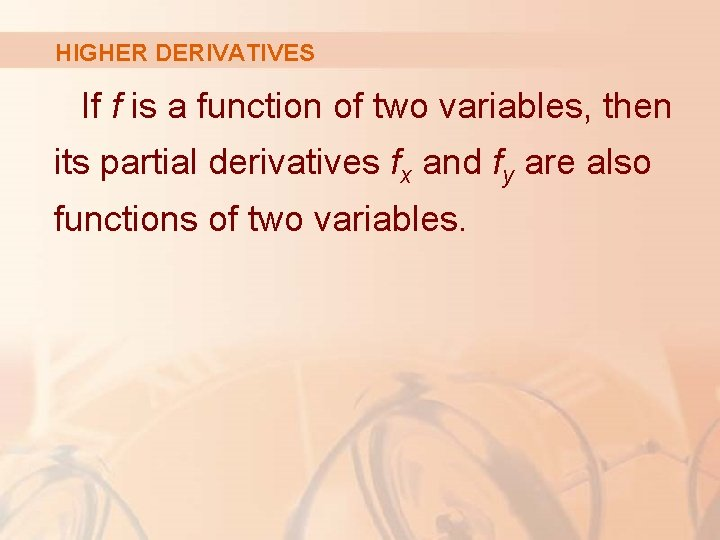HIGHER DERIVATIVES If f is a function of two variables, then its partial derivatives fx and fy are also functions of two variables.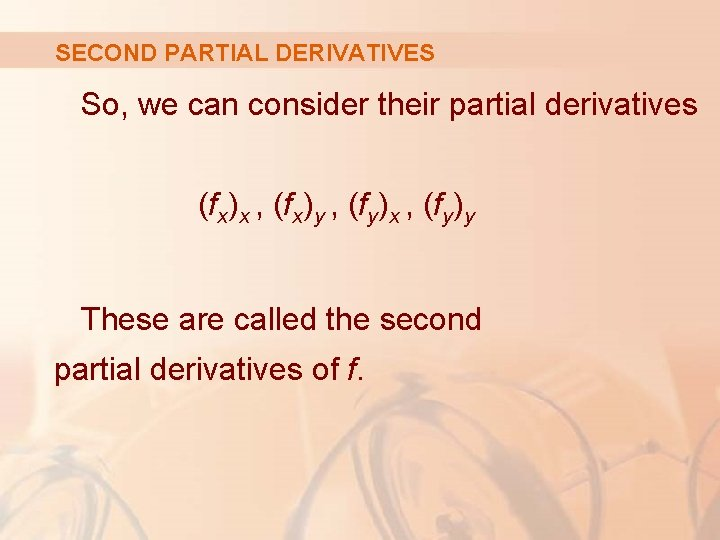SECOND PARTIAL DERIVATIVES So, we can consider their partial derivatives (fx)x , (fx)y , (fy)x , (fy)y These are called the second partial derivatives of f.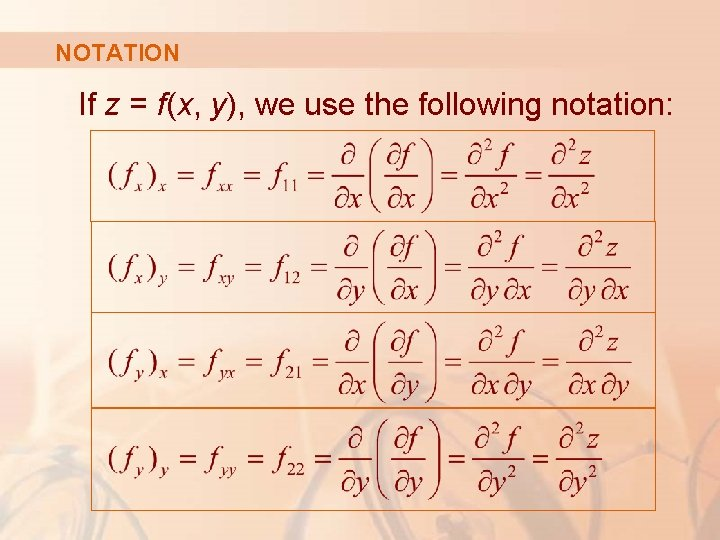NOTATION If z = f(x, y), we use the following notation:SECOND PARTIAL DERIVATIVES Thus, the notation fxy (or ∂2 f/∂y∂x) means that we first differentiate with respect to x and then with respect to y. In computing fyx , the order is reversed.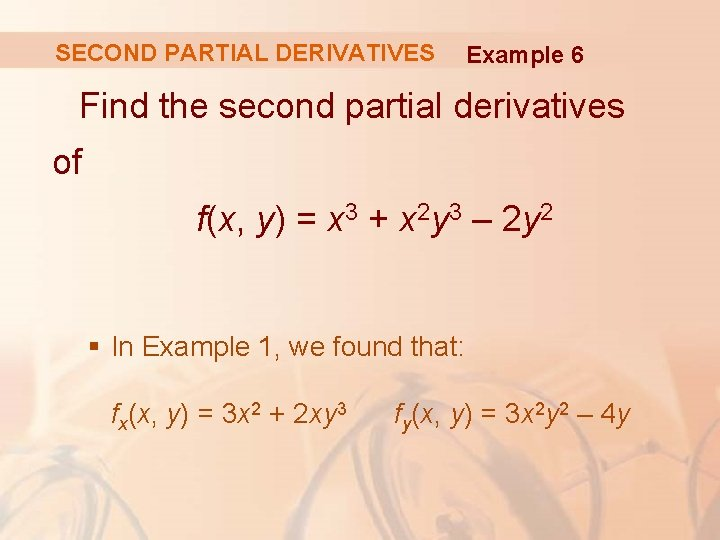SECOND PARTIAL DERIVATIVES Example 6 Find the second partial derivatives of f(x, y) = x 3 + x 2 y 3 – 2 y 2 § In Example 1, we found that: fx(x, y) = 3 x 2 + 2 xy 3 fy(x, y) = 3 x 2 y 2 – 4 ySECOND PARTIAL DERIVATIVES § Hence, Example 6SECOND PARTIAL DERIVATIVES The figure shows the graph of the function f in Example 6 for: – 2 ≤ x ≤ 2, – 2 ≤ y ≤ 2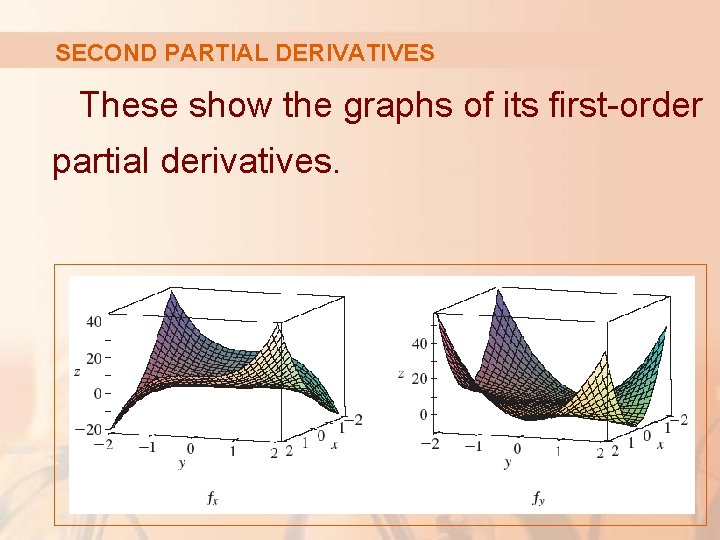SECOND PARTIAL DERIVATIVES These show the graphs of its first-order partial derivatives.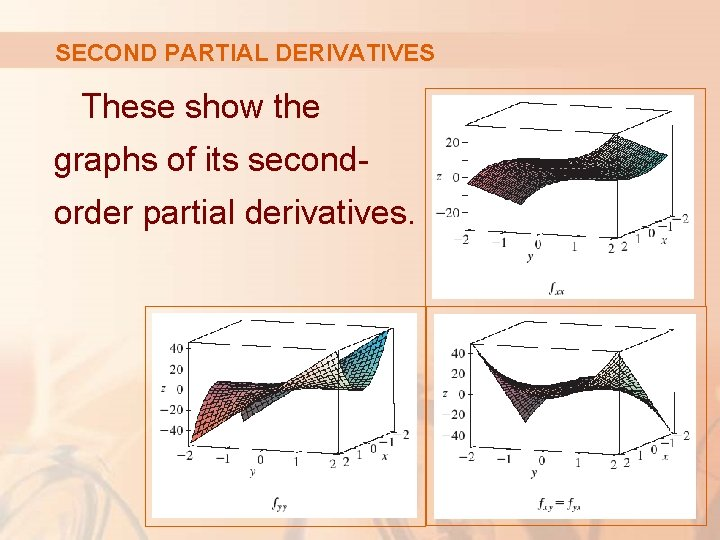SECOND PARTIAL DERIVATIVES These show the graphs of its secondorder partial derivatives.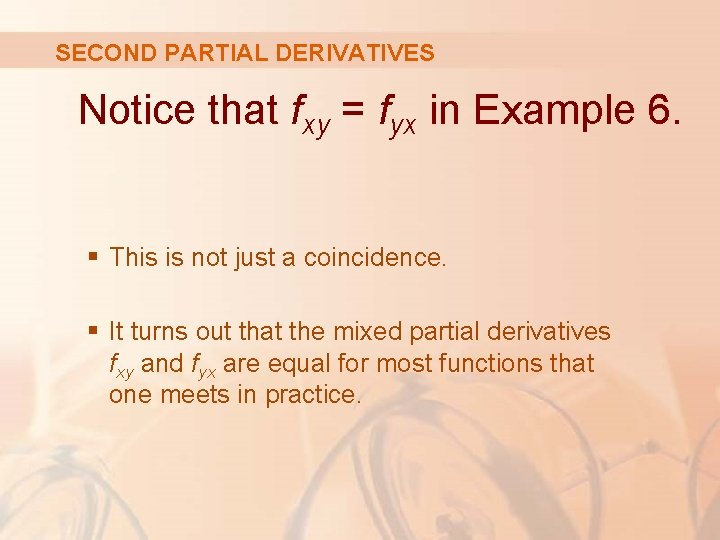SECOND PARTIAL DERIVATIVES Notice that fxy = fyx in Example 6. § This is not just a coincidence. § It turns out that the mixed partial derivatives fxy and fyx are equal for most functions that one meets in practice.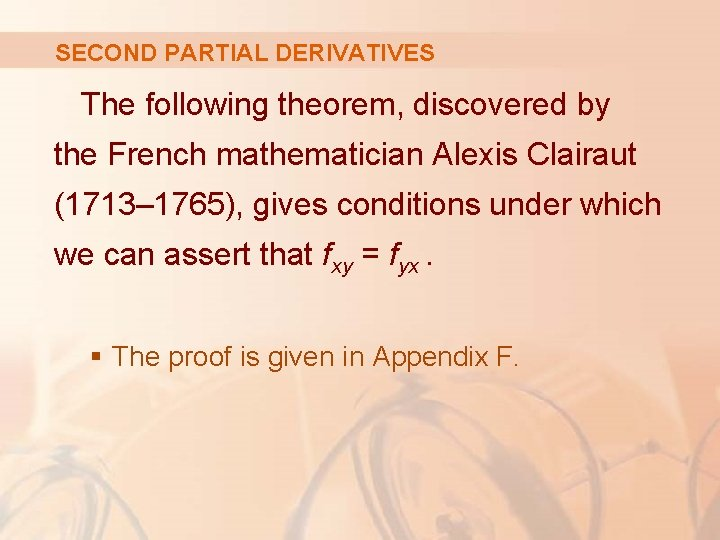SECOND PARTIAL DERIVATIVES The following theorem, discovered by the French mathematician Alexis Clairaut (1713– 1765), gives conditions under which we can assert that fxy = fyx. § The proof is given in Appendix F.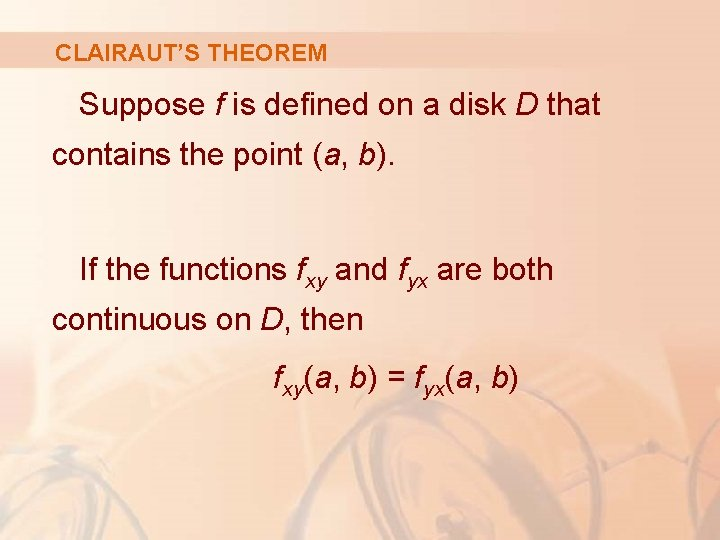CLAIRAUT’S THEOREM Suppose f is defined on a disk D that contains the point (a, b). If the functions fxy and fyx are both continuous on D, then fxy(a, b) = fyx(a, b)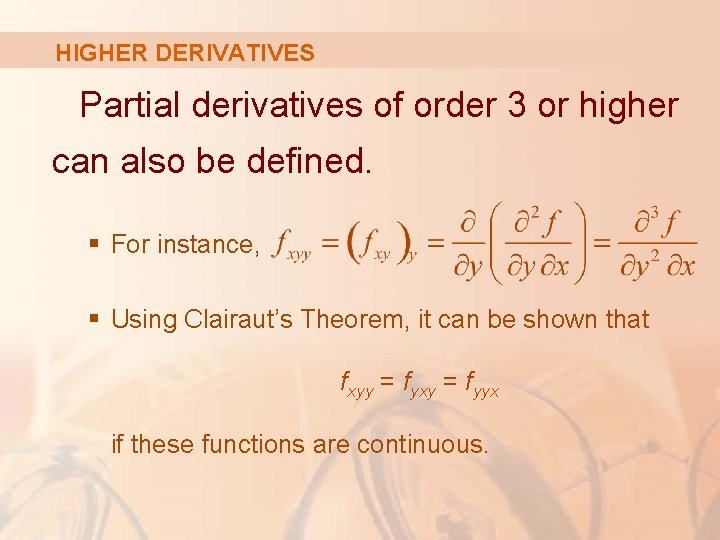HIGHER DERIVATIVES Partial derivatives of order 3 or higher can also be defined. § For instance, § Using Clairaut’s Theorem, it can be shown that fxyy = fyxy = fyyx if these functions are continuous.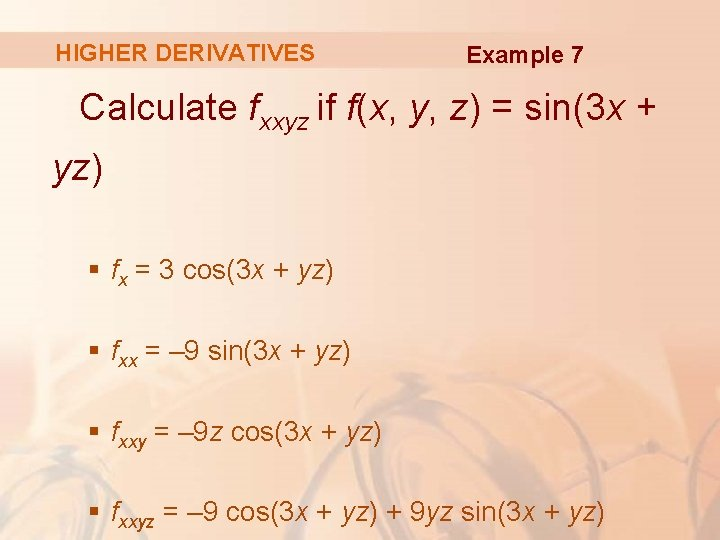HIGHER DERIVATIVES Example 7 Calculate fxxyz if f(x, y, z) = sin(3 x + yz) § fx = 3 cos(3 x + yz) § fxx = – 9 sin(3 x + yz) § fxxy = – 9 z cos(3 x + yz) § fxxyz = – 9 cos(3 x + yz) + 9 yz sin(3 x + yz)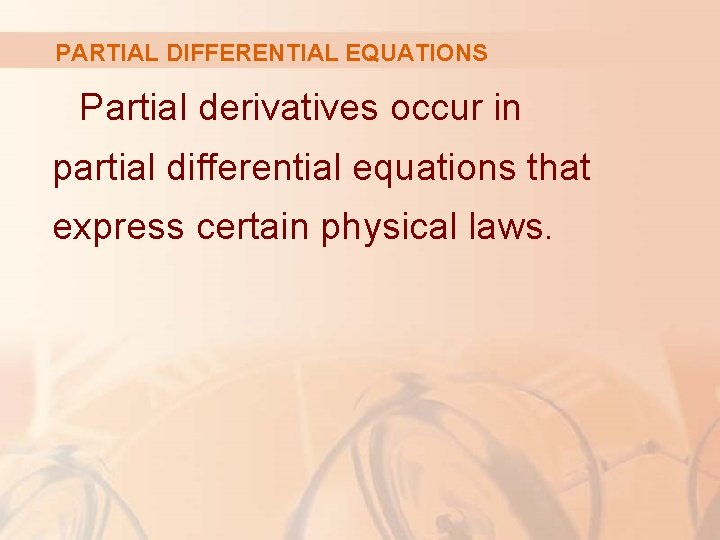PARTIAL DIFFERENTIAL EQUATIONS Partial derivatives occur in partial differential equations that express certain physical laws.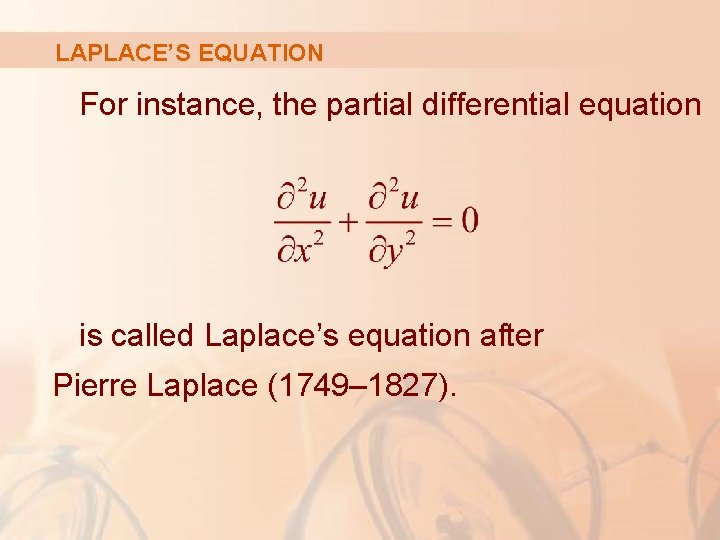LAPLACE’S EQUATION For instance, the partial differential equation is called Laplace’s equation after Pierre Laplace (1749– 1827).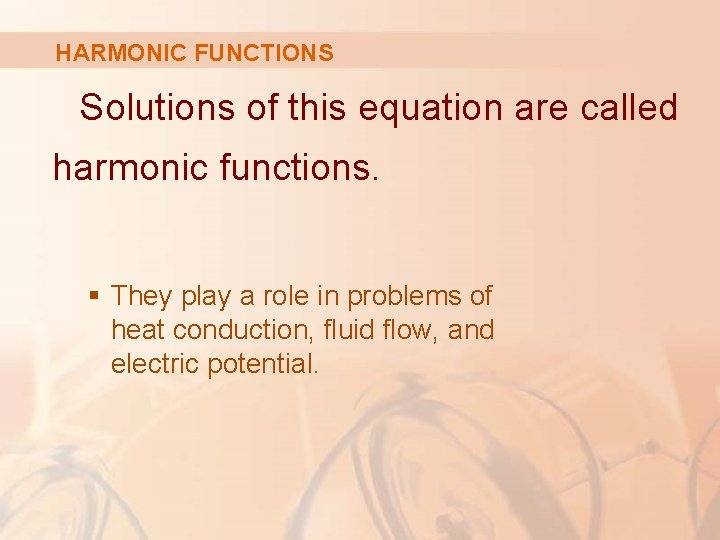HARMONIC FUNCTIONS Solutions of this equation are called harmonic functions. § They play a role in problems of heat conduction, fluid flow, and electric potential.LAPLACE’S EQUATION Example 8 Show that the function u(x, y) = ex sin y is a solution of Laplace’s equation. § § § ux = ex sin y uy = ex cos y uxx = ex sin y uyy = –ex sin y uxx + uyy = ex sin y – ex sin y = 0 § Thus, u satisfies Laplace’s equation.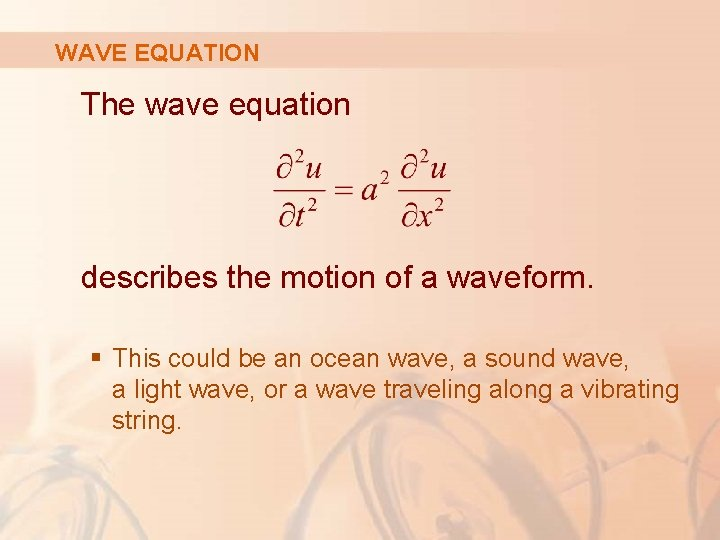WAVE EQUATION The wave equation describes the motion of a waveform. § This could be an ocean wave, a sound wave, a light wave, or a wave traveling along a vibrating string.WAVE EQUATION For instance, if u(x, t) represents the displacement of a vibrating violin string at time t and at a distance x from one end of the string, then § u(x, t) satisfies the wave equation.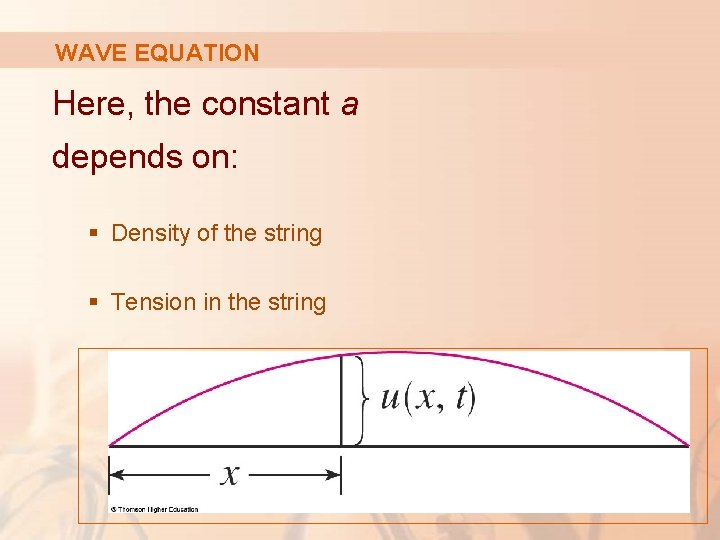WAVE EQUATION Here, the constant a depends on: § Density of the string § Tension in the string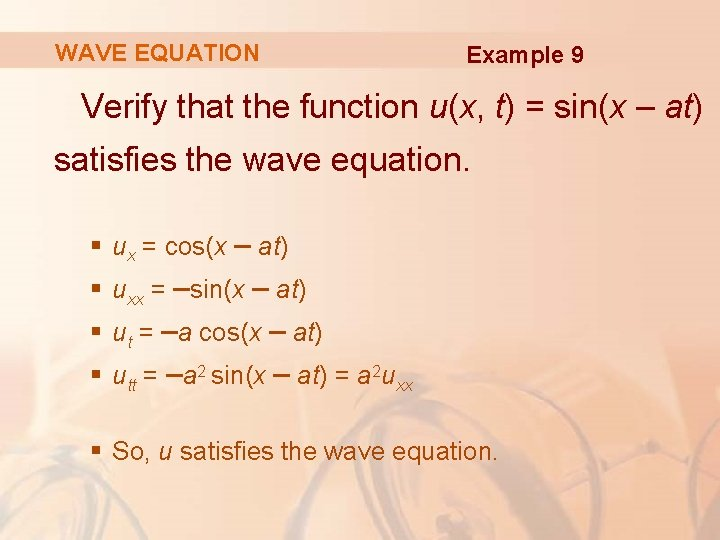WAVE EQUATION Example 9 Verify that the function u(x, t) = sin(x – at) satisfies the wave equation. § ux = cos(x – at) § uxx = –sin(x – at) § ut = –a cos(x – at) § utt = –a 2 sin(x – at) = a 2 uxx § So, u satisfies the wave equation.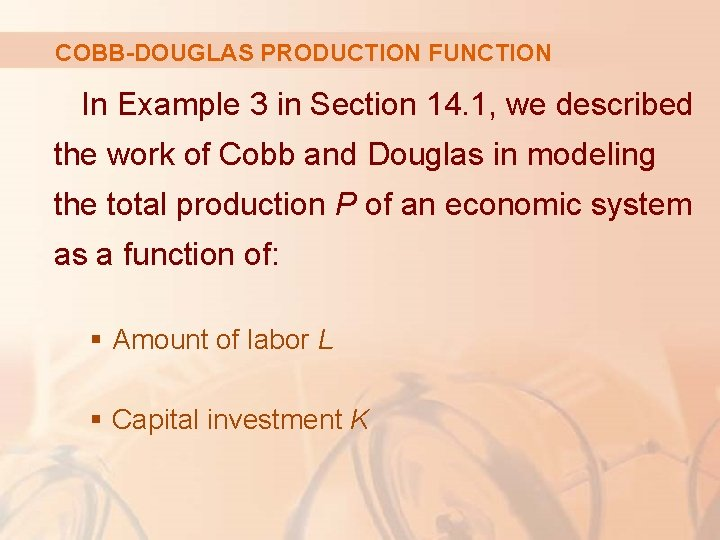COBB-DOUGLAS PRODUCTION FUNCTION In Example 3 in Section 14. 1, we described the work of Cobb and Douglas in modeling the total production P of an economic system as a function of: § Amount of labor L § Capital investment KCOBB-DOUGLAS PRODUCTION FUNCTION Here, we use partial derivatives to show how: § The particular form of their model follows from certain assumptions they made about the economy.MARGINAL PRODUCTIVITY OF LABOR If the production function is denoted by P = P(L, K), then the partial derivative ∂P/∂L is the rate at which production changes with respect to the amount of labor. § Economists call it the marginal production with respect to labor or the marginal productivity of labor.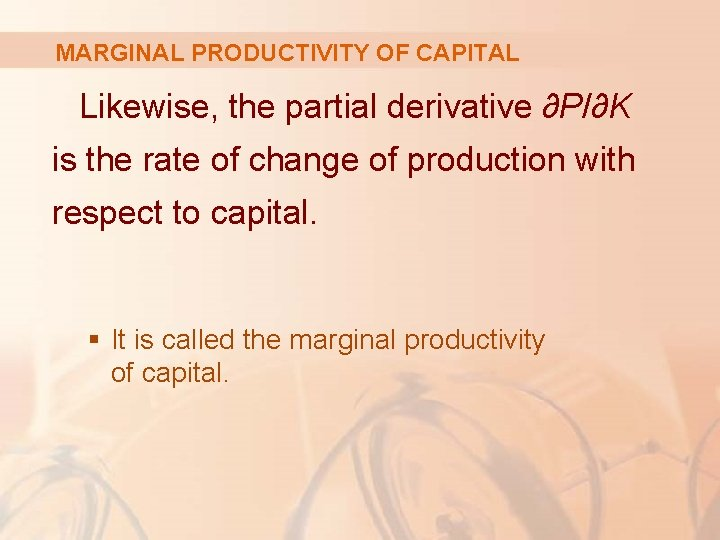MARGINAL PRODUCTIVITY OF CAPITAL Likewise, the partial derivative ∂P/∂K is the rate of change of production with respect to capital. § It is called the marginal productivity of capital.COBB-DOUGLAS ASSUMPTIONS In these terms, the assumptions made by Cobb and Douglas can be stated as follows. i. If either labor or capital vanishes, so will production. ii. The marginal productivity of labor is proportional to the amount of production per unit of labor. iii. The marginal productivity of capital is proportional to the amount of production per unit of capital.COBB-DOUGLAS ASSUMPTION ii Since the production per unit of labor is P/L, assumption ii says that: for some constant α.COBB-DOUGLAS ASSUMPTION ii Equation 5 If we keep K constant (K = K 0), this partial differential equation becomes an ordinary differential equation:COBB-DOUGLAS ASSUMPTION ii Equation 6 If we solve this separable differential equation by the methods of Section 9. 3, we get: P(L, K 0) = C 1(K 0)Lα § Notice that we have written the constant C 1 as a function of K 0 since it could depend on the value of K 0.COBB-DOUGLAS ASSUMPTION iii Similarly, assumption iii says that:COBB-DOUGLAS ASSUMPTION iii Equation 7 We can solve that differential equation to get: P(L 0, K) = C 2(L 0)Kβ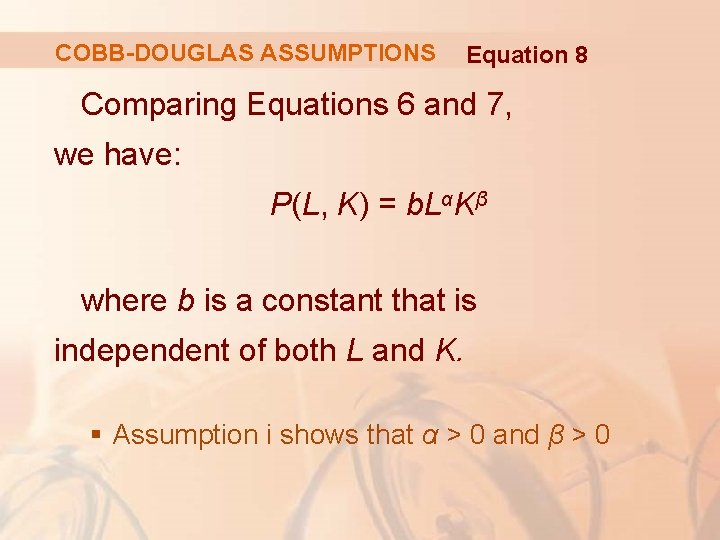COBB-DOUGLAS ASSUMPTIONS Equation 8 Comparing Equations 6 and 7, we have: P(L, K) = b. LαKβ where b is a constant that is independent of both L and K. § Assumption i shows that α > 0 and β > 0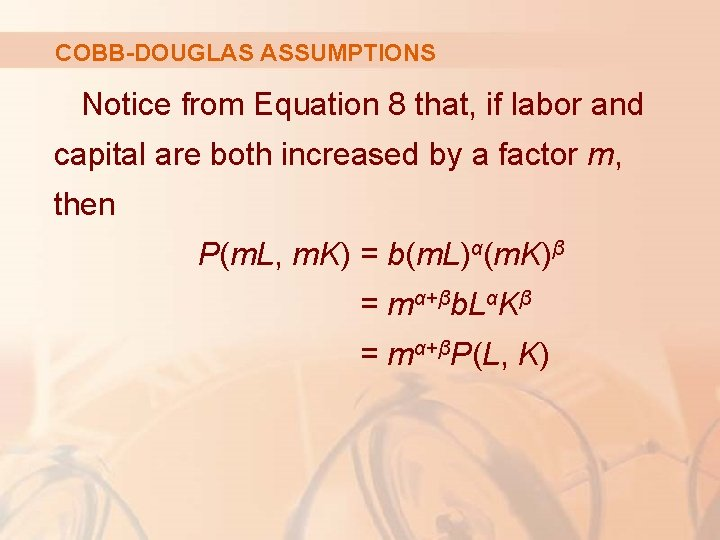COBB-DOUGLAS ASSUMPTIONS Notice from Equation 8 that, if labor and capital are both increased by a factor m, then P(m. L, m. K) = b(m. L)α(m. K)β = mα+βb. LαKβ = mα+βP(L, K)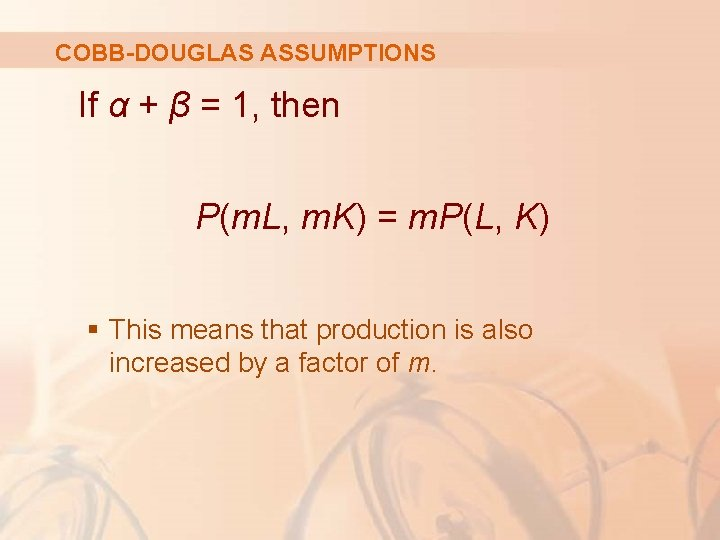COBB-DOUGLAS ASSUMPTIONS If α + β = 1, then P(m. L, m. K) = m. P(L, K) § This means that production is also increased by a factor of m.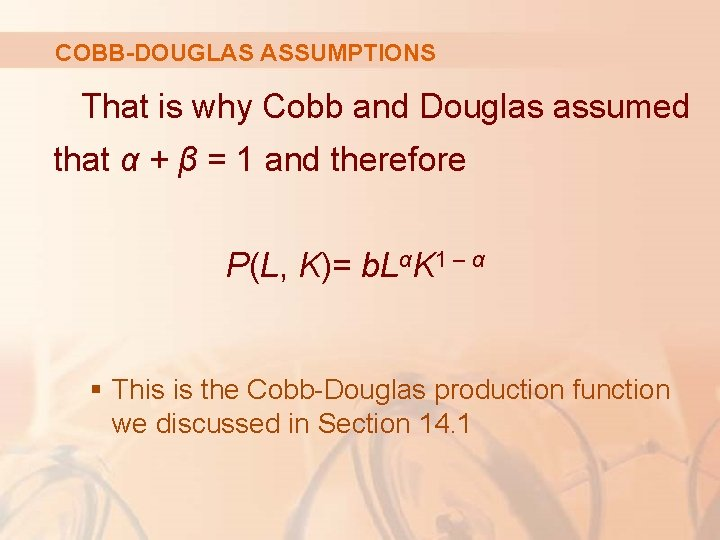COBB-DOUGLAS ASSUMPTIONS That is why Cobb and Douglas assumed that α + β = 1 and therefore P(L, K)= b. LαK 1 – α § This is the Cobb-Douglas production function we discussed in Section 14. 1